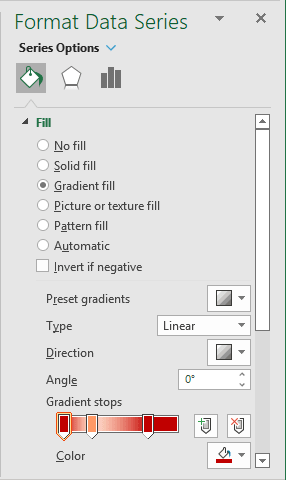# Creating a semaphore thermometer chart

Excel
Data visualization is a powerful cognitive tool that enables you to attract more attention to your statements. E.g., you can direct your organization's attention to insufficiently developed goals and praise them for successfully performed goals. This tip shows you how to update thermometer char colors automatically according to the current process status.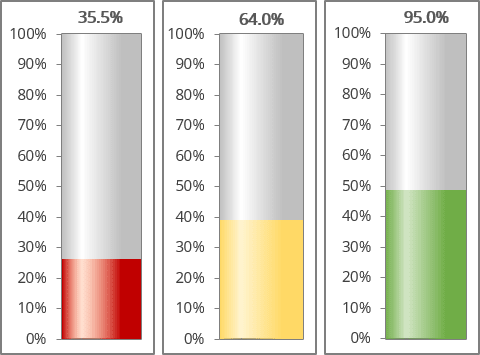For example, a spreadsheet is set up to track daily progress toward a Goal of 1000 new sales in 10 days. Cell C16 contains the Goal value, and cell C17 contains a simple sum formula:

= SUM (C5:C14)

Cell C19 contains a formula that calculates the percent of the Goal (see Creating a simple thermometer chart):

= C17 / C16: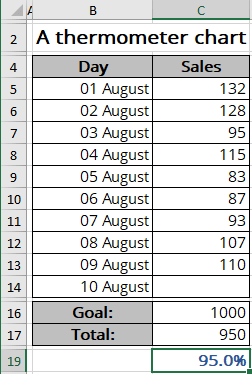To create a chart like the one above, do the following:

In this example, the chart should display different colors for different results achieved:

•   Gray   for achieved results less than 25%:

= IF (<value> < 25%, <value>, 0)

•   Red   if more than 25% but less than 50% is reached:

= IF (IF (<value> < 50%, <value>, 0) > 25%, <value>, 0)

•   Yellow   if an achieved result with a range greater than 50% but less than 75%:

= IF (IF (<value> < 75%, <value>, 0) > 50%, <value>, 0)

•   Green   if the Goal is already closer than 75%:

= IF (<value> > 75%, <value>, 0)

Note: You can use the AND () function instead of two IF () functions. For example:

= IF (AND (<value> < 50%, <value> > 25%), <value>, 0)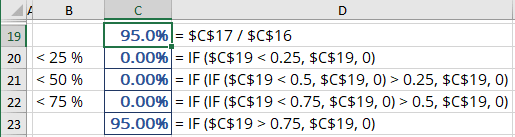### Create a chart

2.   Select the new cells with calculated values (in this example, C20:C23).

3.   On the Insert tab, in the Charts group, click the Insert Bar or Column Chart button: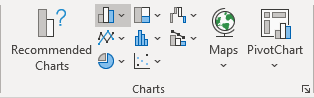Choose Stacked Column: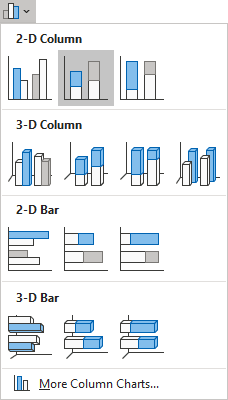### Change the chart data series

By default, Excel creates a column chart from the range of data in one column (one row) as separate columns: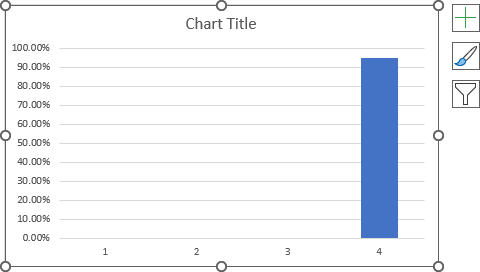If you have a range of data in one row or one column (as in the example), you need to switch columns and rows to display the data correctly on the chart:

4.   Do one of the following:

• Right-click on the chart plot area, then choose Select Data... in the popup menu: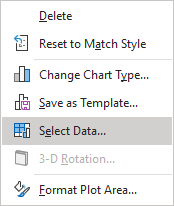In the Select Data Source dialog box, click the Switch Row/Column button: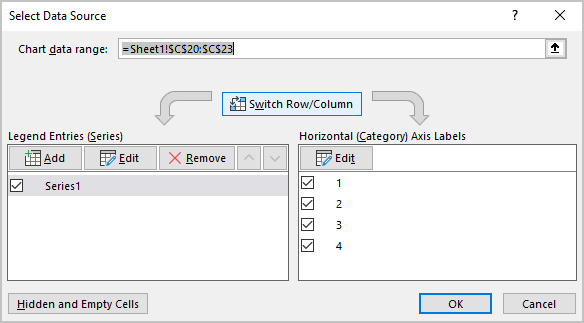• Select the chart, then on the Chart Design tab, in the Data group, click the Switch Row/Column button: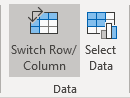Excel rebuilds the chart as needed: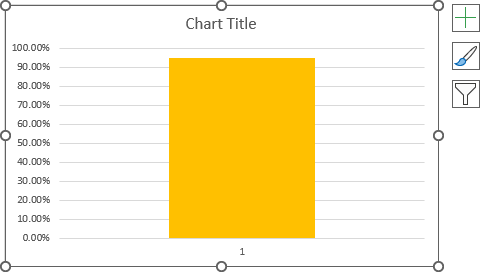### Format the chart

5.   Remove the horizontal (Category) axis and the chart title if necessary.

As you can see, the chart only shows one data series at a time. To set up all possible variants, you need to emulate different variations of the data used for the chart (you will also check the correctness of the used formulas).

So, to customize the first data series, substitute the value of the first range into the source cell (for this example, put a value less than 25% in cell C19).

6.   Right-click on the data series and choose Format Data Series... in the popup menu: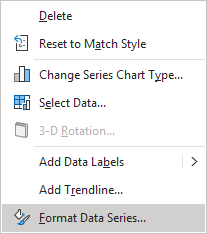On the Format Data Series pane, on the Fill & Line tab, in the Fill section, select one of the existing options (see more about filling options).

For example, select the Gradient fill option, then choose the colors you prefer: